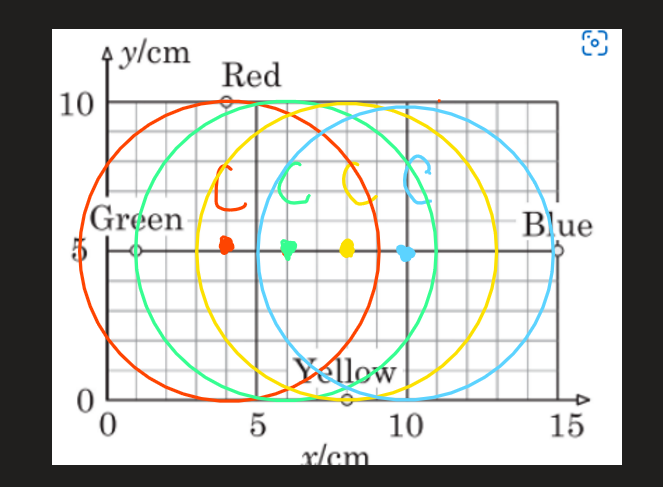# Please solve this mechanics question

Please solve this difficult problem. If anyone knows what is its original source, please do tell me!
Thank you.

1 симпатия

First of all, let’s look at the motion of the disk:
Consider a point A, which is located on the circumference of a disk. We can divide the motion of a point A into o motion of the center and the motion of a point A relative to the center:

Let’s find the center of the disk for each moment (when the lamp emits colored light pulses):
It’s evident that the disk moves along the x-axis and the velocity of the center also lie along this axis. It’s also apparent that the lowest point on the circumference of the disk should have y=0 cm (zero y component), then it’s of course yellow point which has coord. (8,0). We can say that the red(with coord. (4,10)) point is the highest because it has a maximum y component (y= 10 cm). It’s logical and intuitive because the circle (disk) should fit into this surface(XY) (and also the lamp is always in the same place on the circumference of the circle - red, yellow, and other points are the one moving point of disk).
Then if the red is the highest and yellow is the lowest point, the center should be equal distances away from them (symmetry) - 5 cm, then the radius of the disk is also 5 cm (half of the distance between red and yellow points):

Every point on the circumference of the disk is away from the center for a distance R=5cm (radius). Then we can find the position of the center for each light pulses. (if we also know that center moves onle horizontally and its 5 cm away from yellow and red points)The sequence of light pulses : red, green, yellow, blue. Then, looking at the displacements of center:

we can find velocity of center: v=2cm/t=2cm/1s=2cm/s (because time interval t=1s)

For each time interval of 1s lamp rotates around the center of the disk for 90 degrees:

12 симпатий

Thank you so much! It must have taken you a lot of effort and time to describe everything in detail. I really appreciate your help!

2 симпатии

You’re welcome

1 симпатия